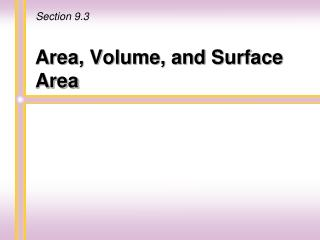DownloadDownload PresentationArea, Volume, and Surface Area

# Area, Volume, and Surface Area

Télécharger la présentation## Area, Volume, and Surface Area

- - - - - - - - - - - - - - - - - - - - - - - - - - - E N D - - - - - - - - - - - - - - - - - - - - - - - - - - -
##### Presentation Transcript

1. Section 9.3 Area, Volume, and Surface Area

2. Area of a Rectangle Areais measured in square units. A square unit is a square one unit on each side. For example, start with a rectangle with length (l) 3 units and width (w) 2 units. A=l•w A= 3•2 units2 A= 6 units2 2 3

3. 2 2 Rectangle 2 3 2 3 3•2=6 A=lw 3 3 (3•2) = 3 1 A=bh 2 Area Formulas Parallelogram Triangle The diagonal of a parallelogram forms 2 congruent triangles. 3•2 = 6 A=bh Martin-Gay, Prealgebra, 5ed

4. B Square h b B b side side h More Area Formulas Trapezoid + area=side •side A=s•s=s2 Martin-Gay, Prealgebra, 5ed

5. Helpful Hint Area is always measured in square units. When finding the area of figures, check to make sure that all measurements are the same units before calculations are made. Martin-Gay, Prealgebra, 5ed

6. r Given a circle of radius, r,the circumference isC= 2r. Martin-Gay, Prealgebra, 5ed

7. Dividing the circle into 4 equal sectors, half the circumference, r, is blue and the other half is red. 8 equal sectors r r r r r r 16 equal sectors 32 equal sectors r r r r r r Area of a Circle Martin-Gay, Prealgebra, 5ed

8. 64 equal sectors 128 equal sectors r r r r r r n 2 equal sectors r r r Area of a Circle Notice the rectangular shape. A = lw A = (r)r A =r2 Martin-Gay, Prealgebra, 5ed

9. Perimeter/ Circumference Plane Figure Drawing Area P=a+ b+c Triangle P=a+b+c+d Parallelogram A=bh P = 2l+ 2w Rectangle A=lw Square P= 4s A=s2 P=a+b+c+d Trapezoid C=d or 2r A=r2 Circle Martin-Gay, Prealgebra, 5ed

10. Volumemeasures the number of cubic units that fill the space of a solid. The volume of a box or can is the amount of space inside. Volume Volume can be used to describe the amount of juice in a pitcher or the amount of concrete needed to pour a foundation for a house. Martin-Gay, Prealgebra, 5ed

11. A polyhedron is a solid formed by the intersection of a finite number of planes. Surface Area The surface area of a polyhedron is the sum of the areas of the faces of the polyhedron. Surface area is measured in square units. Martin-Gay, Prealgebra, 5ed

12. The volume of a solid is the number of cubic units in the solid. 1 inch 1 centimeter 1 centimeter 1 inch 1 centimeter 1 inch 1 cubic centimeter 1 cubic inch Martin-Gay, Prealgebra, 5ed

13. Rectangular Solid height width length Volume =lengthwidth height V=lwh SA = 2lh + 2wh + 2lw Martin-Gay, Prealgebra, 5ed

14. Cube side side side Volume =sidesideside V= s3 SA= 6s3 Martin-Gay, Prealgebra, 5ed

15. Sphere radius Volume = Martin-Gay, Prealgebra, 5ed

16. Circular Cylinder height radius Volume= V=r2h SA= 2rh + 2r2 Martin-Gay, Prealgebra, 5ed

17. Cone height radius Volume =

18. 1 2 side height ( ) · · 3 1 V= s2h 3 Square-Based Pyramid height side Volume = B = area of base, p = perimeter, l = slant height

19. Helpful Hint Volume is always measured in cubic units. Surface area is always measured in square units. Martin-Gay, Prealgebra, 5ed